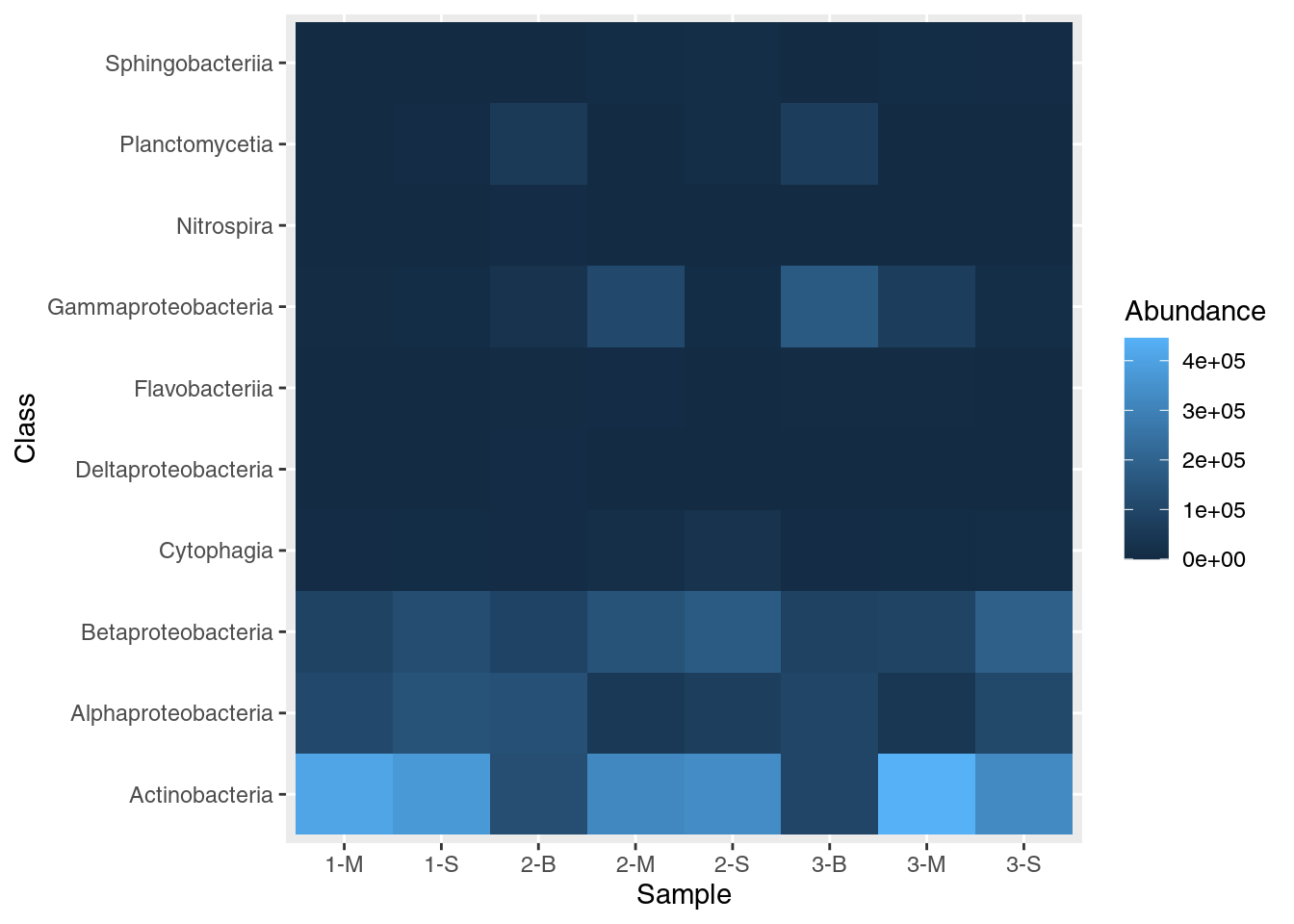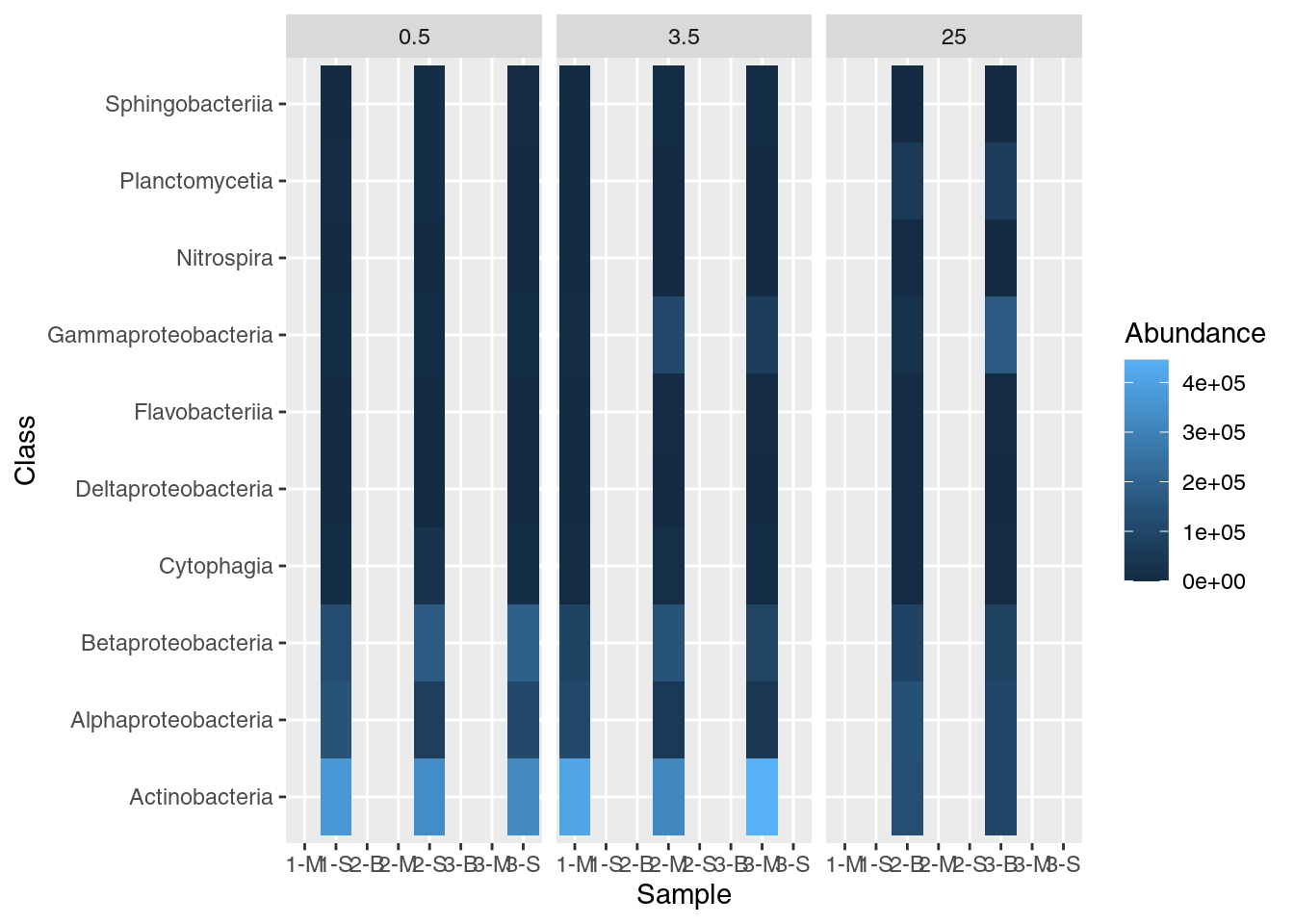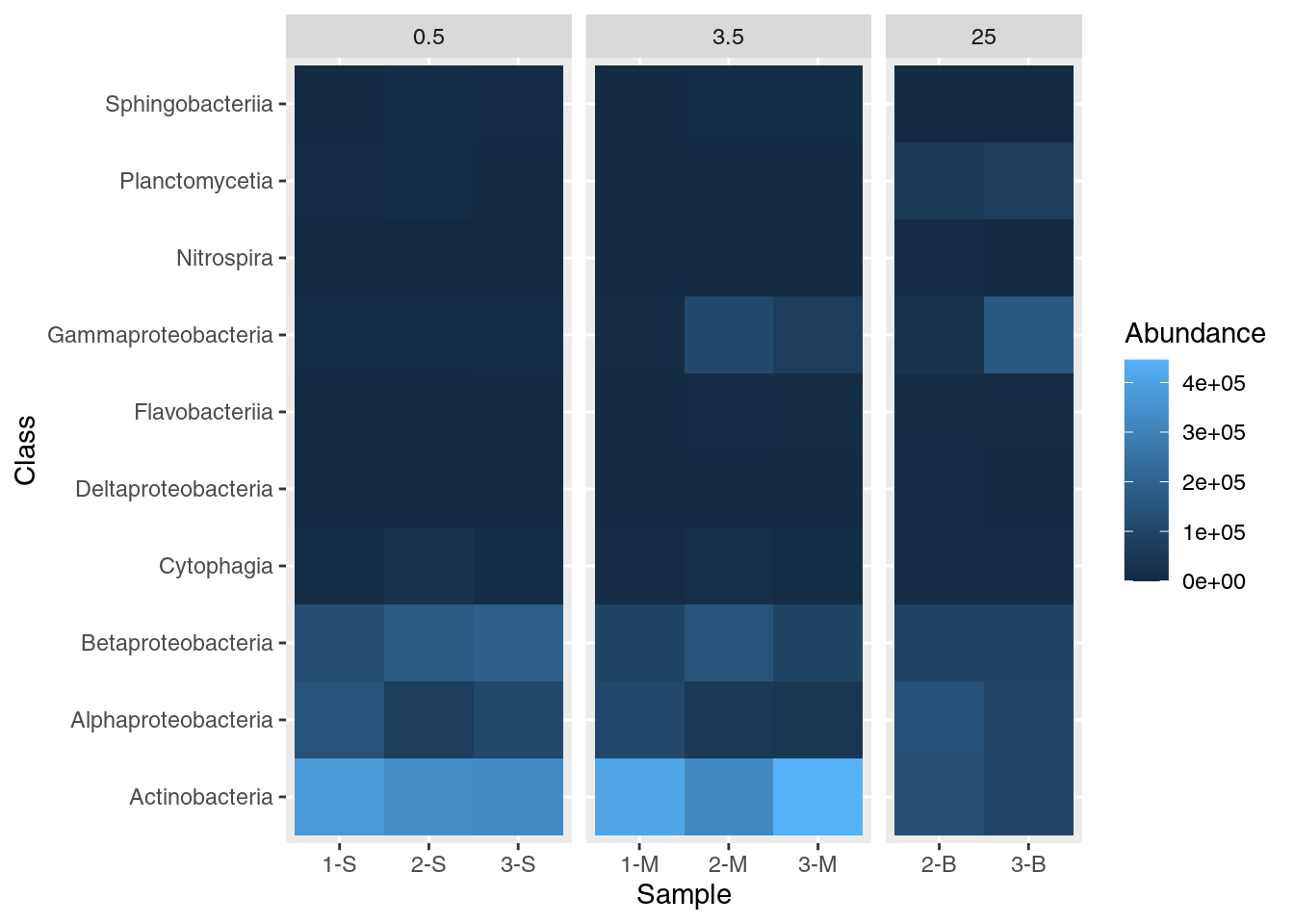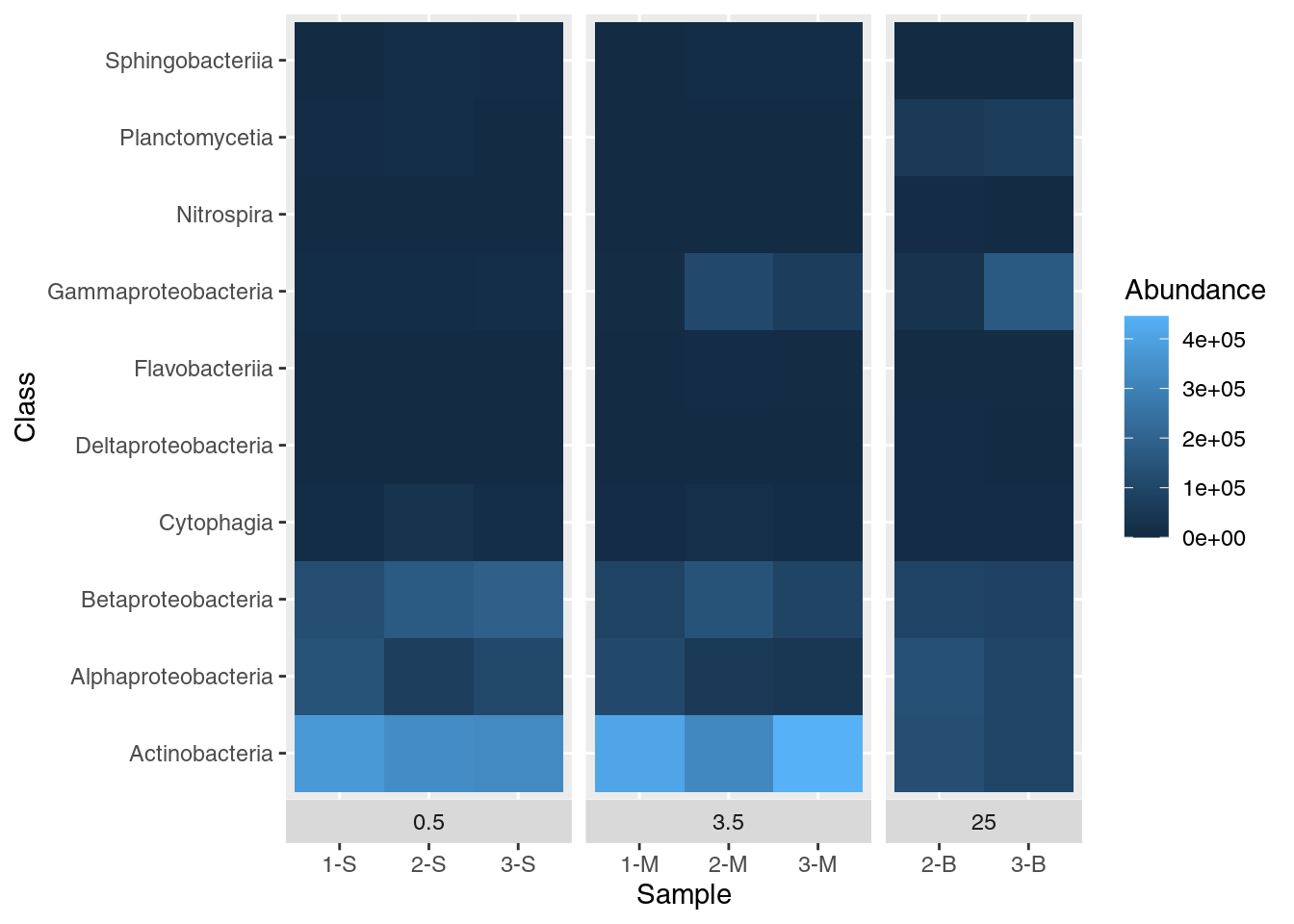A worked example of making heatmaps in R with the `ggplot` package, as well as some data wrangling to easily format the data needed for the plot.

#### Learning objectives

1. Manipulate data into a ‘tidy’ format
2. Visualize data in a heatmap
3. Become familiar with `ggplot` syntax for customizing plots

## Heatmaps & data wrangling

Heatmaps are a great way of displaying three-dimensional data in only two dimensions. But how can we easily translate tabular data into a format for heatmap plotting? By taking advantage of “data munging” and graphics packages, heatmaps are relatively easy to produce in R.

## Getting started

Start by creating a new project in RStudio and creating two folders we’ll use to organize our efforts. The two folders should be `data` and `output` and will store…data and output.

``````dir.create("data")
dir.create("output")``````

For this lesson we will use data from a diversity survey of microbial life in a chrysotile asbestos mine. Driscoll and colleagues doi: 10.1016/j.gdata.2016.11.004 used next generation sequencing to identify and categorize bacterial diversity in a flooded pit of the abandoned mine. The data are normalized read counts for microbes found at the sites. A subset of the data can be downloaded from https://tinyurl.com/mine-data-csv (which itself redirects to https://jcoliver.github.io/learn-r/data/mine-microbe-class-data.csv). You can download this file to your computer with the `download.file` function:

``````download.file(url = "https://tinyurl.com/mine-data-csv",
destfile = "data/mine-data.csv")``````

This downloads the data and saves it in the `data` directory as `mine-data.csv`.

## Data Wrangling

We’ll need to start by reading the data into memory then formatting it for use by the ggplot package. We want all our work to be reproducible, so create a script where we can store all the commands we use to create the heatmap. We begin this script with brief information about the purpose of the script:

``````# Heatmap of mine pit microbe diversity
# Jeff Oliver
# jcoliver@email.arizona.edu
# 2017-06-05``````

Now we read those abundance data into memory:

``mine.data <- read.csv(file = "data/mine-data.csv")``

Take a quick look at the structure of the data, using the `str` command:

``str(mine.data)``
``````## 'data.frame':    8 obs. of  13 variables:
##  \$ Site               : int  1 2 3 1 2 3 2 3
##  \$ Depth              : num  0.5 0.5 0.5 3.5 3.5 3.5 25 25
##  \$ Sample.name        : chr  "1-S" "2-S" "3-S" "1-M" ...
##  \$ Actinobacteria     : int  373871 332856 326695 409809 319778 445572 128251 96304
##  \$ Cytophagia         : int  8052 28561 10468 4481 15885 7302 4732 5566
##  \$ Flavobacteriia     : int  0 0 0 0 5230 6218 5917 6353
##  \$ Sphingobacteriia   : int  0 10013 4918 0 8274 8284 0 0
##  \$ Nitrospira         : int  0 0 0 0 0 0 4609 0
##  \$ Planctomycetia     : int  4553 10008 0 0 0 0 56836 67380
##  \$ Alphaproteobacteria: int  143534 70575 105890 110746 52504 45000 133851 95580
##  \$ Betaproteobacteria : int  124454 170161 187673 87245 146073 91711 90204 85707
##  \$ Deltaproteobacteria: int  0 0 0 0 0 0 4260 0
##  \$ Gammaproteobacteria: int  8426 9005 12935 7025 110825 69452 31956 165572``````

The data frame has 8 rows (“obs.”) and 13 columns (“variables”). The first three columns have information about the observation (Site, Depth, Sample.name), and the remaining columns have the abundance for each of 10 classes of bacteria.

We ultimately want a heatmap where the different sites are shown along the x-axis, the classes of bacteria are shown along the y-axis, and the shading of the cell reflects the abundance. This latter value, the abundance of a bacteria at specific depths at different site, is really just a third dimension. However, instead of creating a 3-dimensional plot that can be difficult to visualize, we instead use shading for our “z-axis”. To this end, we need our data formatted so we have a column corresponding to each of these three dimensions:

• X: Sample identity
• Y: Bacterial class
• Z: Abundance

The challenge is that our data are not formatted like this. While the `Sample.name` column corresponds to what we would like for our x-axis, we do not have columns that correspond to what is needed for the y- and z-axes. All the data are in our data frame, but we need to take a table that looks like this:

Site.name Actinobacteria Cytophagia
1-S 373871 8052
2-S 332856 28561

And transform it to one with a column for bacterial class and a column for abundance, like this:

Site.name Class Abundance
1-S Actinobacteria 373871
1-S Cytophagia 8052
2-S Actinobacteria 332856
2-S Cytophagia 28561

Thankfully, there is an R package that does this for us! The `tidyr` package is designed for creating this type of “tidy”" data.

``install.packages("tidyr")``
``library("tidyr")``

In fact, we can do this transformation in one line with the `pivot_longer` function:

``````mine.long <- pivot_longer(data = mine.data,
cols = everything(),
names_to = "Class",
values_to = "Abundance")``````
``## Error: Can't combine `Site` <double> and `Sample.name` <character>.``

Uh oh. That error is preventing us from transforming our data to long format. What R is telling us is that we tried to create a column with multiple data types in it, which is a big no-no in R. That is, it attempted to make a single column that had both the data from the “Site” column (which are numbers) with the data from the “Sample.name” column, which is a factor (factors are what R calls categories).

Consider our original data structure:

``str(mine.data)``
``````## 'data.frame':    8 obs. of  13 variables:
##  \$ Site               : int  1 2 3 1 2 3 2 3
##  \$ Depth              : num  0.5 0.5 0.5 3.5 3.5 3.5 25 25
##  \$ Sample.name        : chr  "1-S" "2-S" "3-S" "1-M" ...
##  \$ Actinobacteria     : int  373871 332856 326695 409809 319778 445572 128251 96304
##  \$ Cytophagia         : int  8052 28561 10468 4481 15885 7302 4732 5566
##  \$ Flavobacteriia     : int  0 0 0 0 5230 6218 5917 6353
##  \$ Sphingobacteriia   : int  0 10013 4918 0 8274 8284 0 0
##  \$ Nitrospira         : int  0 0 0 0 0 0 4609 0
##  \$ Planctomycetia     : int  4553 10008 0 0 0 0 56836 67380
##  \$ Alphaproteobacteria: int  143534 70575 105890 110746 52504 45000 133851 95580
##  \$ Betaproteobacteria : int  124454 170161 187673 87245 146073 91711 90204 85707
##  \$ Deltaproteobacteria: int  0 0 0 0 0 0 4260 0
##  \$ Gammaproteobacteria: int  8426 9005 12935 7025 110825 69452 31956 165572``````

Notice there are columns that don’t need to be transformed for our heatmap. Namely, Site, Depth, and Sample.name can all remain as-is. To do this, we instruct `pivot_longer` to ignore the first three columns of the data frame by negating those columns (`-c(1:3`):

``````mine.long <- pivot_longer(data = mine.data,
cols = -c(1:3),
names_to = "Class",
values_to = "Abundance")
``````## # A tibble: 6 x 5
##    Site Depth Sample.name Class            Abundance
##   <int> <dbl> <chr>       <chr>                <int>
## 1     1   0.5 1-S         Actinobacteria      373871
## 2     1   0.5 1-S         Cytophagia            8052
## 3     1   0.5 1-S         Flavobacteriia           0
## 4     1   0.5 1-S         Sphingobacteriia         0
## 5     1   0.5 1-S         Nitrospira               0
## 6     1   0.5 1-S         Planctomycetia        4553``````

Another approach, to accomplish the same thing, especially if the columns to ignore are not adjacent, is to explicitly name the columns to ignore:

``````mine.long <- pivot_longer(data = mine.data,
cols = -c(Site, Depth, Sample.name),
names_to = "Class",
values_to = "Abundance")``````

To recap, at this point we read in the data and transformed it for easy use with heatmap tools:

``````# Heatmap of mine pit microbe diversity
# Jeff Oliver
# jcoliver@email.arizona.edu
# 2017-06-05

library("tidyr")

# Read data and format for heatmap
mine.long <- pivot_longer(data = mine.data,
cols = -c(1:3),
names_to = "Class",
values_to = "Abundance")``````

Now the data are ready - on to the plot!

## Plotting heatmaps

### The ggplot package

To plot a heatmap, we are going to use the `ggplot2` package.

``install.packages("ggplot2")``
``library("ggplot2")``

For this plot, we are going to first create the heatmap object with the `ggplot` function, then print the plot. We create the object by assigning the output of the `ggplot` call to the variable `mine.heatmap`, then entering the name of this object to print it to the screen.

``````mine.heatmap <- ggplot(data = mine.long, mapping = aes(x = Sample.name,
y = Class,
fill = Abundance)) +
geom_tile() +
xlab(label = "Sample")

mine.heatmap``````Let’s dissect these commands:

• `ggplot` is the initial call, where we provide the following information:
• `data` is the data frame that contains the data we want to plot
• `mapping` tells ggplot what to plot where; that is, in this call, it says we want the `Sample.name` column on the x-axis, the bacterial `Class` on the y-axis, and the shading, or fill, (the z-axis) to reflect the value in the `Abundance` column.
• `geom_tile`, which is appended to `ggplot` with a plus sign (+), tells ggplot that we want a heatmap
• `xlab` provides a label to use for the x-axis

This command follows the commonly used syntax for creating ggplot objects, where an initial call to `ggplot` sets up preliminary plotting information, and details are appended with plus signs:

``````# Create plot object
plot.object.name <- ggplot(data, mapping) +
layer.one() +
layer.two() +
layer.three()

# Draw plot
plot.object.name``````

### Faceting a plot

The ggplot package includes a great way to visualize different categories of data. This approach, called “faceting” requires one additional layer to the plotting command called `facet_grid`. With `facet_grid` we indicate which column contains the categories we want to use for the plot:

``````mine.heatmap <- ggplot(data = mine.long, mapping = aes(x = Sample.name,
y = Class,
fill = Abundance)) +
geom_tile() +
xlab(label = "Sample") +
facet_grid(~ Depth)

mine.heatmap``````But this leaves a bit to be desired. We only want columns displayed for which there are data, so we have to add some additional information in our `facet_grid` call:

``````mine.heatmap <- ggplot(data = mine.long, mapping = aes(x = Sample.name,
y = Class,
fill = Abundance)) +
geom_tile() +
xlab(label = "Sample") +
facet_grid(~ Depth, scales = "free_x", space = "free_x")

mine.heatmap``````• Passing `"free_x"` to the `scales` argument instructs R to allow different x-axis for each of our sub-plots. In this case, the default is for each site to appear in each sub-plot. Specifying `scales = "free_x"` removes any site from a sub-plot where there is no corresponding data. That is, it removes sites 1-S, 2-S, and 3-S from the 3.5 and 25 depth sub-plots, removes 1-M, 2-M, and 3-M from the 0.5 and 25 depth sub-plots, and removes 2-B and 3-B from the 0.5 and 3.5 depth sub-plots.
• Passing `"free_x"` to `space` ensures that each column in the plot is the same width. Try it without specifying the `space` argument to see the effect of this behavior.

While we are here, we can also move the boxes labelling each sub-plot (0.5, 3.5, and 25) from the top of the plot to the bottom of the plot with the `switch` argument in the `facet_grid()` function:

``````mine.heatmap <- ggplot(data = mine.long, mapping = aes(x = Sample.name,
y = Class,
fill = Abundance)) +
geom_tile() +
xlab(label = "Sample") +
facet_grid(~ Depth, switch = "x", scales = "free_x", space = "free_x")

mine.heatmap``````That’s better. There is still some room for improvement, but we’ll get to that later.

### Improving aesthetics

#### Scale

First let’s deal with a scaling issue. Most values are dark blue, but this is partly caused by the distribution of values - there are a few really high values in the Abundance column. We can transform the data for display by taking the square root of abundance and plotting that. To do this, we need to add another column to our data frame and update our call to ggplot to reference this new column, `Sqrt.abundance`:

``````mine.long\$Sqrt.abundance <- sqrt(mine.long\$Abundance)
mine.heatmap <- ggplot(data = mine.long, mapping = aes(x = Sample.name,
y = Class,
fill = Sqrt.abundance)) +
geom_tile() +
xlab(label = "Sample") +
facet_grid(~ Depth, switch = "x", scales = "free_x", space = "free_x")

mine.heatmap``````